Thank you for visiting nature.com. You are using a browser version with limited support for CSS. To obtain the best experience, we recommend you use a more up to date browser (or turn off compatibility mode in Internet Explorer). In the meantime, to ensure continued support, we are displaying the site without styles and JavaScript.

Activity driven modeling of time varying networks

Abstract

Network modeling plays a critical role in identifying statistical regularities and structural principles common to many systems. The large majority of recent modeling approaches are connectivity driven. The structural patterns of the network are at the basis of the mechanisms ruling the network formation. Connectivity driven models necessarily provide a time-aggregated representation that may fail to describe the instantaneous and fluctuating dynamics of many networks. We address this challenge by defining the activity potential, a time invariant function characterizing the agents' interactions and constructing an activity driven model capable of encoding the instantaneous time description of the network dynamics. The model provides an explanation of structural features such as the presence of hubs, which simply originate from the heterogeneous activity of agents. Within this framework, highly dynamical networks can be described analytically, allowing a quantitative discussion of the biases induced by the time-aggregated representations in the analysis of dynamical processes.

Introduction

Network modeling1,2,3,4,5,6 has long drawn on the tradition of social network analysis and graph theory, with models ranging from the Erdös-Rényi model to Logit models, p*-models and Markov random graphs7,8,9,10,11. In the last decade, the class of growing network models, exemplified by the preferential attachment model, has been made widely popular by research in statistical physics and computer science12,13,14,15,16,17. All these models can be defined as connectivity driven, as the network's topology is at the core of the model's algorithmic definition. Connectivity-driven network models are well-suited for capturing the essential features of systems such as the Internet, where connections among nodes are long-lived elements18,19. However, in many cases the interactions among the elements of the system are rapidly changing and are characterized by processes whose timing and duration are defined on a very short time scale20,21. This limit has been investigated in the case of adaptive systems whose structure evolve being coupled to the process taking place on top of them22,23,24,25,26. Instead, the understating of this limit in time varying networks in which the structure evolves independently of the process is still limited and unexplored. In these activity-driven networks, models intended to capture the process of accumulating connections over time and the resulting degree distribution (i.e. the probability that a node has k connections to other nodes) and other topological properties merely represent a time-integrated perspective of the system. Furthermore, the analysis of dynamical processes in evolving networks is generally performed in the presence of a time-scale separation between the network evolution and the dynamical process unfolding on its structure. In one limit we can consider the network as quenched in its connectivity pattern, thus evolving on a time scale that is much longer that the dynamical process itself. In the other limiting case, the network is evolving at a time scale much shorter than the dynamical process thus effectively disappearing from the definition of the interaction among individuals that is conveniently replaced by effective random couplings. While time scale separation is extremely convenient for the numerical and analytical tractability of the models, networks generally evolve on a time-scale that might be comparable to the one of the dynamical process27,28,29,30,31,32. An accurate modelization of the dynamics of activity-driven networks calls for the definition of interaction processes based on the actual measurement of the activity of the agents forming the system, a task now made possible by the availability of time-resolved, high-quality data on the instantaneous activity of millions of agents in a wide variety of networks33,34,35,36,37.

Results

Here we present the analysis of three large-scale, time-resolved network datasets and define for each node a measurable quantity, the activity potential, characterizing its interaction pattern within the network. This measure is defined as the number of interactions performed, in a given time window, by each node divided by the total number of interactions made by all the nodes in the same time window. We find that the system level dynamics of the network can be encoded by the activity potential distribution function from which it is possible to derive the appropriate interaction rate among nodes. On the basis of the empirically measured activity potential distribution we propose a process model for the generation of random dynamic networks. The activity potential function defines the network structure in time and traces back the origin of hubs to the heterogenous activity of the network elements. The model allows to write dynamical equations coupling the network dynamics and the dynamical processes unfolding on its structure without relying on any time-scale separation approximation. We analyze a simple spreading process and provide the explicit analytical expression for the biases introduced by the time-aggregated representation of the network when studying dynamical processes occurring on a time scale comparable to that of the network evolution. Interestingly, the network model presented here is amenable to the introduction of many features in the nodes' dynamic such as the the persistency of specific interactions or assortative/disassortative correlations, thus defining a general basic modeling framework for rapidly evolving networks.

The activity potential

We consider three datasets corresponding to networks in which we can measure the individual agents' activity: Collaborations in the journal “Physical Review Letters” (PRL) published by the American Physical Society38, messages exchanged over the Twitter microblogging network and the activity of actors in movies and TV series as recorded in the Internet Movie Database (IMDb)39. In the first dataset the network representation considers undirected links connecting two PRL authors if they have collaborated in writing one article. In the second system each node is a Twitter user and an undirected link is drawn if at least one message has been exchanged between two users. Finally, the actor network is obtained by drawing an undirected link between any two actors who have participated in the same movie or TV series.

Simple evidence for the role of agents' activity in network analysis and modeling can be readily observed in the case of the collaboration network of scientific authors40. The number of collaborations of any author depends on the time window through which we observe the system. In Fig. 1 we show the networks obtained by time-aggregated co-authorships over 1, 10 and 30 years for the subset of authors in the PRL dataset who were active in the considered time period. Clearly, the time scale used to construct the network defines a non-stationary connectivity pattern and explicitly affects the network structure and its degree distribution. Similar results are found for the other two datasets as shown in the Supplementary Information.

In the three datasets considered, we characterize the individual activity of every agent: papers written, messages exchanged, or movie appearances, respectively. For each dataset we measure the individual activity of each agent and define the activity potential xi of the agent i as the number of interactions that he/she performs in a characteristic time window of given length Δt, divided by the total number of interactions made by all agents during the same time window. The activity potential xi thus estimates the probability that the agent i was involved in any given interaction in the system and the probability distribution F(x) that a randomly chosen agent i has activity potential x statistically defines the interaction dynamics of the system. In Fig. 2 we show the cumulative distribution Fc(x) evaluated for the three datasets. In all cases we find that, contrary to the degree distribution and other structural characteristics of the networks, the distribution Fc(x) is virtually independent of the time scale over which the activity potential is measured. Additionally, we find that the distribution Fc(x) is skewed and fairly broadly distributed. This is hardly surprising as in many cases measurements of human activity have confirmed the presence of wide variability across individuals41,42.

Activity driven network model

Our empirical analysis naturally leads to the definition of a simple model that uses the activity distribution to drive the formation of a dynamic network. We consider N nodes (agents) and assign to each node i an activity/firing rate ai = ηxi, defined as the probability per unit time to create new contacts or interactions with other individuals, where η is a rescaling factor defined such that the average number of active nodes per unit time in the system is η〈xN. The activity rates are defined such that the numbers xi are bounded in the intervaland are assigned according to a given probability distribution F(x) that may be chosen arbitrarily or given by empirical data. We impose a lower cut-offon x in order to avoid possible divergences of F(x) close to the origin. We assume a simple generative process according to the following rules (see Fig. 2-D):

• At each discrete time step t the network Gt starts with N disconnected vertices;

• With probability aiΔt each vertex i becomes active and generates m links that are connected to m other randomly selected vertices. Non-active nodes can still receive connections from other active vertices;

• At the next time step t + Δt, all the edges in the network Gt are deleted. From this definition it follows that all interactions have a constant duration τi = Δt.

The above model is random and Markovian in the sense that agents do not have memory of the previous time steps. The full dynamics of the network and its ensuing structure is thus completely encoded in the activity potential distribution F(x).

In Fig. 3 we report the results of numerical simulations of a network with N = 5000, m = 2, η = 10 and F(x) x−γ, with γ = 2.8 and. The model recovers the same qualitative behavior observed in Fig. 1. At each time step the network is a simple random graph with low average connectivity. The accumulation of connections that we observe by measuring activity on increasingly larger time slices T generates a skewed PT(k) degree distribution with a broad variability. The presence of heterogeneities and hubs (nodes with a large number of connections) is due to the wide variation of activity rates in the system and the associated highly active agents. However, it is worth remarking that hub formation has a different interpretation than in growing network prescriptions, such as preferential attachment. In those cases hubs are created by a positional advantage in degree space leading to the passive attraction of more and more connections. In our model, the creation of hubs results from the presence of nodes with high activity rate, which are more willing to repeatedly engage in interactions.

The model allows for a simple analytical treatment. We define the integrated networkGt as the union of all the networks obtained in each previous time step. The instantaneous network generated at each time t will be composed of a set of slightly interconnected nodes corresponding to the agents that were active at that particular time, plus those who received connections from active agents. Each active node will create m links and the total edges per unit time are Et = mNηx〉 yielding the average degree per unit time the contact rate of the network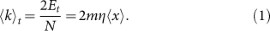The instantaneous network will be composed by a set of stars, the vertices that were active at that time step, with degree larger than or equal to m, plus some vertices with low degree. The corresponding integrated network, on the other hand, will generally not be sparse, being the union of all the instantaneous networks at previous times (see Fig. 3). In fact, for large time T and network size N, when the degree in the integrated network can be approximated by a continuous variable, we can show (see Supplementary Information) that agent i will have at time T a degree in the integrated network given by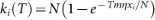. It can then easily be shown that the degree distribution PT(k) of the integrated network at time T takes the form: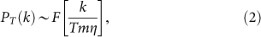where we have considered the limit of small k/N and k/T (i.e. large network size and times). The noticeable result here is the relation between the degree distribution of the integrated network and the distribution of individual activity, which, from the previous equation, share the same functional form. This relation is approximately recovered in the empirical data, where the activity potential distribution is in reasonable agreement with the appropriately rescaled asymptotic degree distribution of the corresponding network (see Fig. 4-A). As expected, differences between the two distributions are present, due to features of the real network dynamics that our random model does not capture: links might have memory (already explored connections are more likely to happen again), social relations have a lifetime distribution (persistence) and multiple connections and weighted links may be relevant. Neither of these effects is considered in the model. We report some statistical analysis of those features in the Supplementary Information as further ingredients to be considered in future extensions of the model.

Dynamical processes in activity driven networks

Recent research has highlighted the key role of interaction dynamics as opposed to static studies. For example, an individual who appears to be central by traditional network metrics may in fact be the last to be infected because of the timing of his/her interactions30,43. Analogously the concurrency of sexual partners can dramatically accelerate the spread of STDs31. Despite its simplicity, our model makes it analytically explicit that the actors' activity time scale plays a major role in the understanding of processes unfolding on dynamical networks. Let us consider the susceptible-infected-susceptible (SIS) epidemic compartmental model1,2,44,45. In this model, infected individuals can propagate the disease to healthy neighbors with probability λ, while infected individuals recover with rate µ and become susceptible again. In an homogenous population the behavior of the epidemics is controlled by the reproductive number R0 = β/µ, where β = λ〈k〉 is the per capita spreading rate that takes into account the rate of contacts of each individual. The reproductive number identifies the average number of secondary cases generated by a primary case in an entirely susceptible population and defines the epidemic threshold such that only if R0 > 1 can epidemics reach an endemic state and spread into a closed population. In the past few years the inclusion of complex connectivity networks and mobility schemes into the substrate of spreading processes contagion, diffusion, transfer, etc. has highlighted new and interesting results46,47,48,49,50. Several results states that the epidemic threshold depends on the topological properties of the networks. In particular, for networks characterized by a fix, quenched topology the threshold is given by the principal eigenvalue of the adjacency matrix48,49. Instead, for annealed network, characterized by a topology defined just on average because the connectivity patterns has a dynamic extremely fast with respect to the dynamical process, heterogeneous mean-field approaches2,6 predict an epidemic threshold that is inversely proportional to the second moment of the network's degree distribution: β/µ > 〈k2/〈k2〉. However, these results do not apply to the case in which the time variation of the connectivity pattern is occurring on the same time scale of the dynamical process. Our model presents simple evidence of this problem, as a disease with a small value of µ−1 (the infectious period characteristic time) will have time to explore the fully-integrated network, but will not spread on the dynamic instantaneous networks whose union defines the integrated one30,31,43,51. In Fig. 4-B we plot the results of numerical simulations of the SIS model on a network generated according to our model and on two time-aggregated network instances. We observe that the two aggregated networks lead to misleading results in both the threshold and the epidemic magnitude as a function of β/µ. Even if the epidemic threshold discounts the different average degree of the networks in the factor β = λ〈k〉, the two aggregated instances consider all edges as always available to carry the contagion process, disregarding the fact that the edges may be active or not according to a specific time sequence defined by the agents' activity.

The above finding can be more precisely quantified by calculating analytically the epidemic threshold in activity driven networks without relying on any time aggregated view of the network connectivity. By working with activity rates we can derive epidemic evolution equation in which the spreading process and the network dynamics are coupled together. Let us assume a distribution of activity potential x of nodes given by a general distribution F(x) as before. At a mean-field level, the epidemic process will be characterized by the number of infected individuals in the class of activity rate a, at time t, namely. The number of infected individuals of class a at time t + Δt given by: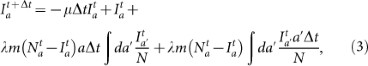where Na is the total number of individuals with activity a. In Eq. (3), the third term on the right side takes into account the probability that a susceptible of class a is active and acquires the infection getting a connection from any other infected individual (summing over all different classes), while the last term takes into account the probability that a susceptible, independently of his activity, gets a connection from any infected active individual. The above equation can be solved as shown in the material and methods section, yielding the following epidemic threshold for the activity driven model:This result considers the activity rate of each actor and therefore takes into account the actual dynamics of interactions; the above formula does not depend on the time-aggregated network representation and provides the epidemic threshold as a function of the interaction rate of the nodes. This allows to characterize the spreading condition on the natural time scale of the combination of the network and spreading process evolution.

Discussion

We have presented a model of dynamical networks that encodes the connectivity pattern in a single function, the activity potential distribution, that can be empirically measured in real world networks for which longitudinal data are available. This function allows the definition of a simple dynamical process based on the nodes' activity rate, providing a time dependent description of the network's connectivity pattern. Despite its simplicity, the model can be used to solve analytically the co-evolution of the network and contagion processes and characterize quantitatively the biases generated by time-scale separation techniques. Furthermore the proposed model appears to be suited as a testbed to discuss the effect of network dynamics on other processes such as damage resilience, discovery and data mining, collective behavior and synchronization. While we have reduced the level of realism for the sake of parsimony of the presented model, we are aware of the importance of analyzing other features of actor activity such as concurrency, persistence and different weights associated with each connection. These features must necessarily be added to the model in order to remove the limitations set by the simple random network structures generated here and represent interesting challenges for future work in this area.

Methods

Datasets

We considered three different dataset: the collaborations in the journal “Physical Review Letters” (PRL) published by the APS, the message exchanged on Twitter and the activity of actors in movies and TV series as recorded in the Internet Movie Database (IMDb). In particular:

PRL dataset

In this database the network representation considers each author of a PRL article as a node. An undirected link between two different authors is drawn if they collaborated in the same article. We filter out all the articles with more than 10 authors in order to focus our attention just on small collaborations in which we can assume that the social components is relevant. We consider the period between 1960 and 2004. In this time window we registered 71, 583 active nodes and 261, 553 connections among them. In this dataset is natural defining the activity rate, a, of each author as the number of papers written in a specific time window Δt = 1 year. Authors with no collaborative papers in the total time span considered (isolates) are not included in the data set.

Having been granted temporary access to Twitter's firehose we mined the stream for over 6 months to identify a large sample of active user accounts. Using the API, we then queried for the complete history of 3 million users, resulting in a total of over 380 million individual tweets covering almost 4 years of user activity on Twitter. In this database the network representation considers each users as a node. An undirected link between two different users is drawn if they exchanged at least one message. We focus our attention on 9 months during 2008. In this time window we registered 531, 788 active nodes and 2, 566, 398 connections among them. In this dataset we define the activity rate of each user as the number of messages sent in a time window Δt = 1 day.

IMDb Dataset

In this database the network representation considers each actor as a node. An undirected link between two different actors is drawn if they collaborated in the same movie/TV series. We focus on the period between 1950 and 2010. During this time period we registered 1, 273, 631 active nodes and 47, 884, 882 connections between them. A natural way to define the activity rate in this dataset is to consider the number of movies acted by each actor in a specific time window Δt = 1 year.

Epidemic threshold

In order to solve Eq. (3) we can consider the total number of infectious nodes in the system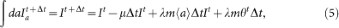whereand we have dropped all second order terms in the activity rate a and in. We are not considering events in which two infected nodes choose each other for connection and we are considering a linear approximation insince in the beginning of the epidemics the number of infectious individuals in each class is small. In order to obtain an closed expression for θ we multiply both sides of Eq. (3) by a and integrate over all activity spectrum, obtaining the equation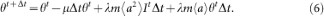In the continuous time limit we obtain the following closed system of equations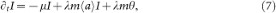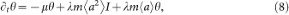whose Jacobian matrix has eigenvalues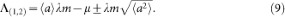The epidemic threshold for the system is obtained requiring the largest eigenvalues to be larger the 0, which leads to the condition for the presence of an endemic state: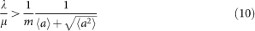From this last expression we can recover the epidemic threshold of Eq. (4) by considering β = λ〈k〉, ai = ηxi and 〈k〉 = 2x〉.

References

1. Newman, M. Networks. An Introduction (Oxford Univesity Press, 2010).

2. Barrat, A., Barthélemy, M. & Vespignani, A. Dynamical Processes on Complex Networks (Cambridge Univesity Press, 2008).

3. Albert, R. & Barabási, A.-L. Statistical mechanics of complex networks. Rev. Mod. Phys. 74, 47 (2002).

4. Boccaletti, S., Latora, V., Moreno, Y., Chavez, M. & Hwang, D.-U. Complex networks: Structure and dynamics. Physics Reports 424, 175 (2006).

5. Bollobas, B. Moder Graph Theory (Springer-Verlag, 1998).

6. Vespignani, A. Modeling dynamical processes in complex socio-technical systems. Nature Physics 8, 32–39 (2012).

7. Erdös, P. & Rényi, A. On random graphs. Publications mathematicae 6, 290 (1959).

8. Molloy, M. & Reed, B. A critical point for random graphs with a given degree sequence. Random Structures and Algorithms 6, 161 (1995).

9. Holland, P. & Leinhardt, S. An exponential family of probabilty distributions of directed graphs. J. Am. Stat. Assoc. 76, 33–50 (1981).

10. Frank, O. & Strauss, D. Markov graphs. J. Am. Stat. Assoc. 81, 832–842 (1986).

11. Wasserman, S. & Pattiston, P. Logit models and logistic regression for social networks. Psychometrika 61, 401–425 (1996).

12. Barabási, A.-L., Albert, R. & Jeong, H. Mean-field theory for scale-free random networks. Physica A 272, 173 (1999).

13. Barabási, A.-L. & Albert, R. Emergence of scaling in random networks. Science 286, 509 (1999).

14. Dorogovtsev, S. N., Mendes, J. & Samukhin, A. N. Structure of growing networks with preferential linking. Phys. Rev. Lett. 85, 4633 (2000).

15. Dorogovtsev, S. N. & Mendes, J. F. F. Evolution of Networks: From Biological nets to the Internet and WWW (2003).

16. Fortunato, S., Flammini, A. & Menczer, F. Scale-free network growth by ranking. Phys. Rev. Lett. 96, 218701 (2006).

17. Boguña, M. & Pastor-Satorras, R. Class of correlated random networks with hidden variables. Phys. Rev. E 68, 036112 (2011).

18. Pastor-Satorras, R. & Vespignani, A. Evolution and Structure of the Internet : A Statistical Physics Approach (Cambridge University Press, 2004).

19. Albert, R., Jeong, H. & Barabási, A.-L. The diameter of the world wide web. Nature 401, 130–131 (1999).

20. Holme, P. & Saramäki, J. Temporal networks. arxiv:1108.1780 (2011).

21. Ghoshal, G. & Holme, P. Attractiveness and activity in internet communities. Physica A 364, 603–609 (2005).

22. Volz, E. & Meyers, L. A. Epidemic thresholds in dynamic contact networks. J. R. Soc. Interface 6, 233241 (2009).

23. Centola, D., Gonzalez-Avella, J. C., Eguiluz, V. M. & San Miguel, M. Homophily, cultural drift and the co-evolution of cultural groups. J. Conflict Resolution 51, 905929 (2007).

24. Jolad, S., Liu, W., Schmittmann, B. & Zia, R. K. P. Epidemic spreading on preferred degree adaptive networks. arxiv:1109.5440 (2011).

25. Schwartz, I. & Shaw, L. Rewiring for adaptation. Physics 3, 17 (2010).

26. Shaw, L. B. & Schwartz, I. B. Enhanced vaccine control of epidemics in adaptive networks. Phys. Rev. E 81, 046120 (2010).

27. Butts, C. Revisting the foundations of network analysis. Science 325, 414–416 (2009).

28. Butts, C. Relational event framework for social action. Sociological Methodology 38, 155–200 (2008).

29. Panisson, A. et al. On the dynamics of human proximity for data diffusion in ad-hoc networks. Ad Hoc Networks (2011).

30. Moody, J. The importance of relationship timing for diffusion: Indirect connectivity and std infection risk. Soc. Forces 81, 25 (2002).

31. Morris, M. & Kretzschmar, M. Concurrent partnerships and the spread of hiv. AIDS 11, 641 (1997).

32. Basu, P., Guha, S., Swami, A. & Towsley, D. Percolation phenomena in networks under random dynamics. In: COMSNETS 2012, Bangalore, India (2012).

33. González, M. C., Hidalgo, C. A. & Barabási, A.-L. Understanding individual human mobility patterns. Nature 453, 779 (2008).

34. Onnela, J.-P. et al. Structure and tie strengths in mobile communication networks. Proc. Natl. Acad. Sci. U.S.A. 104, 7332 (2007).

35. Lazer, D. et al. Computational social science. Science 323, 721 (2009).

36. Vespignani, A. Predicting the behavior of techno-social systems. Science 325, 425–428 (2009).

37. Brockmann, D., Hufnagel, L. & Geisel, T. The scaling laws of human travel. Nature 439, 462 (2006).

38. APS. Data sets for research (2010).

39. IMDb. Internet movie database http://www.imdb.com/interfaces (2010)Date of access 10/11/2011.

40. Newman, M. E. J. The structure of scientific collaboration networks. Proc. Natl. Acad. Sci. 98, 404 (2001).

41. Barabási, A.-L. The origin of bursts and heavy tails in human dynamics. Nature 435, 207 (2005).

42. Jo, H.-H., Karsai, M., Kertész, J. & Kaski, K. Circadian pattern and burstiness in human communication activity. New. J. Phys 14, 013055 (2012).

43. Isella, L. et al. What's in a crowd? analysis of face-to-face behavioral networks. J. Theor. Biol. 271, 166–180 (2011).

44. Kermack, W. O. & McKendrick, A. G. A contribution to the mathematical theory of epidemics. Proc. R. Soc. A 115, 700 (1927).

45. Keeling, M. & Rohani, P. Modeling Infectious Disease in Humans and Animals (Princeton University Press, 2008).

46. Lloyd, A. & May, R. How viruses spread among computers and people. Science 292, 1316 (2001).

47. Balcan, D. et al. Multiscale mobility networks and the spatial spreading of infectious diseases. Proc. Natl. Acad. Sci. U.S.A. 106, 21484 (2009).

48. Wang, Y., Chakrabarti, D., Wang, C. & Faloutsos, C. Epidemic spreading in real networks: An eigenvalue viewpoint. 22nd Symposium on Reliable Distributed Computing (SRDS2003) (2003).

49. Chakrabarti, D., Wang, Y., Wang, C., Leskovec, J. & Faloutsos, C. Epidemic thresholds in real networks. ACM Transactions on Information and System Security (TISSEC), 10 (4) (2008).

50. Castellano, C. & Pastor-Satorras, R. Thresholds for epidemic spreading in networks. Phys. Rev. Lett. 105, 218701 (2010).

51. Morris, M. Sexually Transmitted Diseases, K.K. Holmes, et al. Eds. (McGraw-Hill, 2007).

Acknowledgements

The work has been partly sponsored by the Army Research Laboratory and was accomplished under Cooperative Agreement Number W911NF-09-2-0053. RPS acknowledges financial support from the Spanish MICINN (project FIS2010-21781-C02-01) and additional support through ICREA Academia, funded by the Generalitat de Catalunya.

Author information

Authors

Contributions

R.P.-S. & A.V designed research, N.P. performed simulations, N.P., B.G, R.P.-S. & A.V. analyzed the data, N.P., R.P.-S. & A.V. contributed new analytical results. All authors wrote, reviewed and approved the manuscript.

Ethics declarations

Competing interests

The authors declare no competing financial interests.

Electronic supplementary material

Supplementary Information

Supplementary information

Rights and permissions

Reprints and Permissions

Perra, N., Gonçalves, B., Pastor-Satorras, R. et al. Activity driven modeling of time varying networks. Sci Rep 2, 469 (2012). https://doi.org/10.1038/srep00469

• Accepted:

• Published:

• DOI: https://doi.org/10.1038/srep00469

• Stochastic sampling effects favor manual over digital contact tracing

• Marco Mancastroppa
• Claudio Castellano
• Raffaella Burioni

Nature Communications (2021)

• Emergence and evolution of social networks through exploration of the Adjacent Possible space

• Enrico Ubaldi
• Raffaella Burioni
• Francesca Tria

Communications Physics (2021)

• Spreading of performance fluctuations on real-world project networks

• Iacopo Pozzana
• Christos Ellinas
• Konstantinos Sakellariou

Applied Network Science (2021)

• Human migration: the big data perspective

• Alina Sîrbu
• Rajesh Sharma

International Journal of Data Science and Analytics (2021)

• UTLDR: an agent-based framework for modeling infectious diseases and public interventions

• Giulio Rossetti
• Letizia Milli
• Virginia Morini

Journal of Intelligent Information Systems (2021)